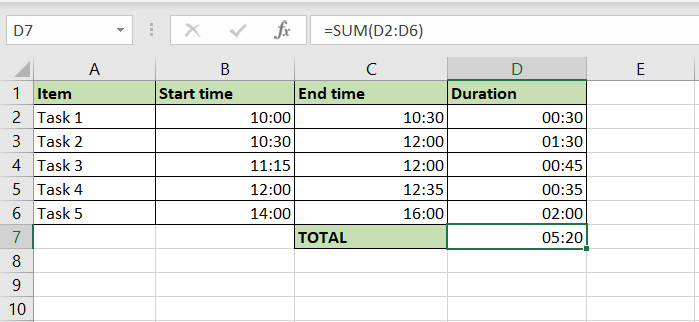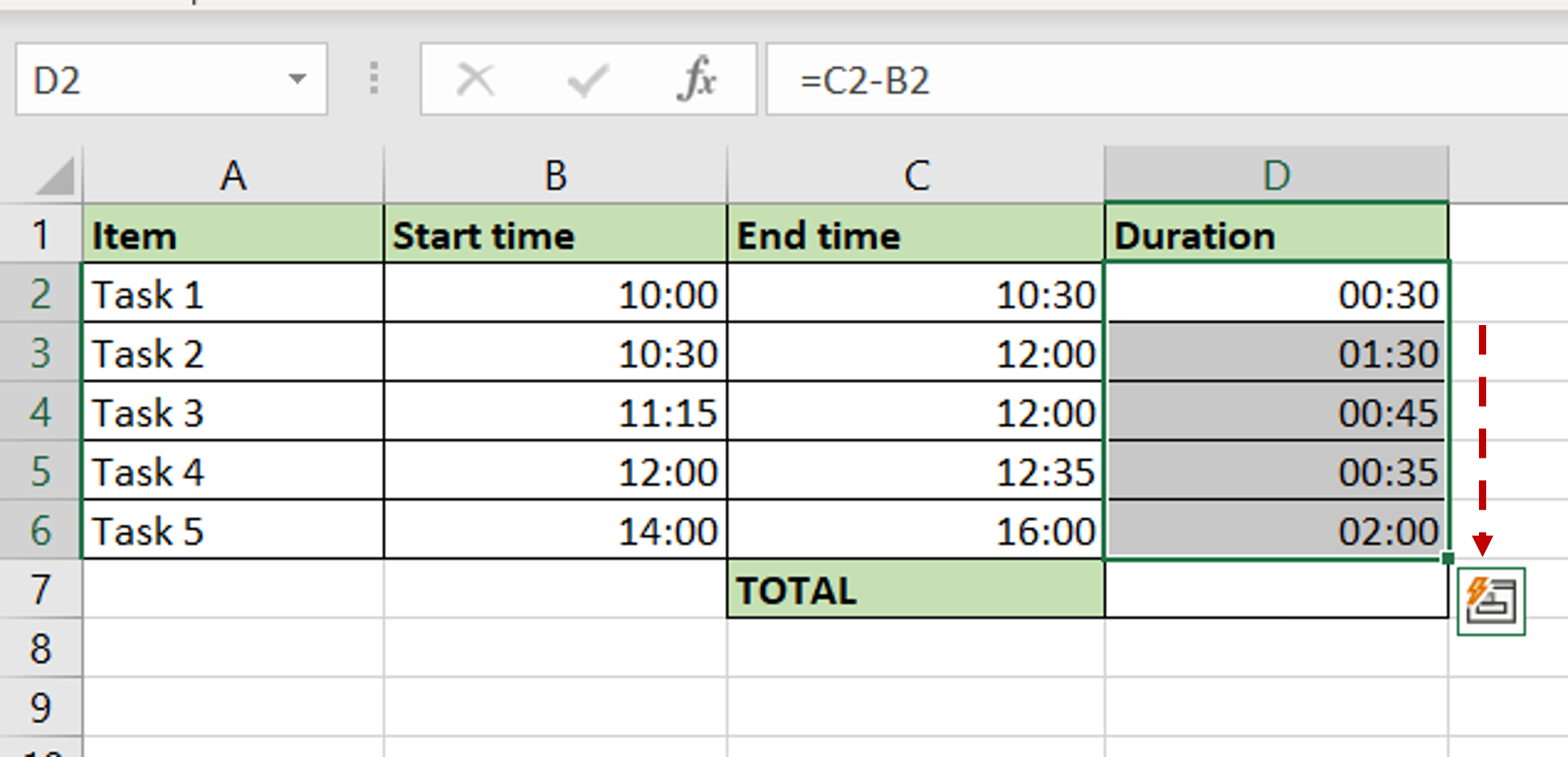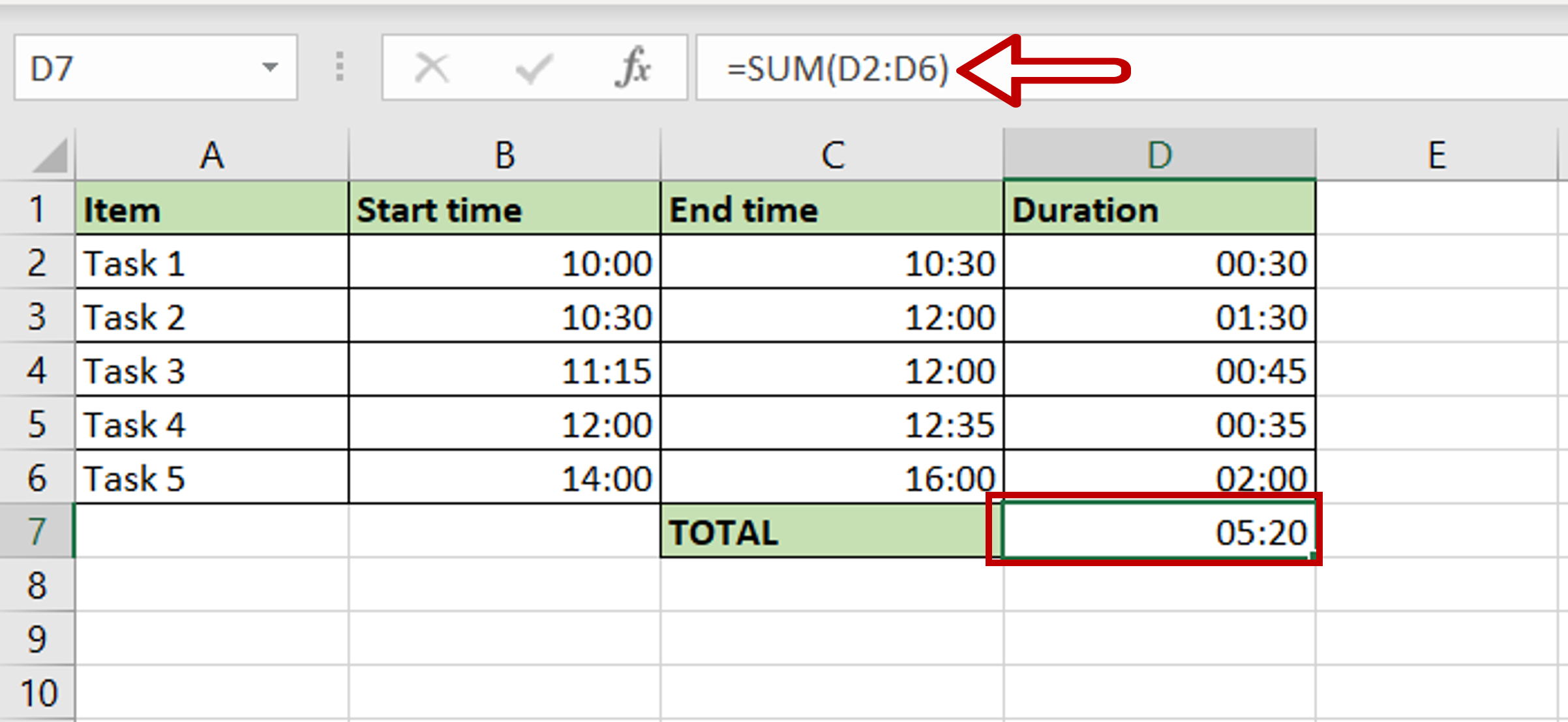# How to add and subtract time in Excel

You can watch a video tutorial here.Excel treats date and time formats differently from numbers and text. Because of the different scales i.e. 24 hours, 60 minutes, and 60 seconds, when adding minutes and seconds they cannot be treated like regular numbers. In Excel, to be able to add or subtract dates and times, you need to format them as dates and times for the operation to work correctly.

### Step 1 – Create the formula to subtract time– Select the cell where the result is to appear
– Type the formula using cell references:
=End time – Start time

### Step 2 – Copy the formula– Using the fill handle from the first cell, drag the formula to the remaining cells
OR
a) Select the cell with the formula and press Ctrl+C or choose Copy from the context menu (right-click)
b) Select the rest of the cells in the column and press Ctrl+V or choose Paste from the context menu (right-click)

### Step 3 – Create the formula to add time– Select the cell where the result is to appear
– Type the formula using cell references:
=SUM(Duration)

### Step 4 – Check the result– Start and End times are subtracted to get the duration of each task
– The time of Duration is added to get the total duration in hours